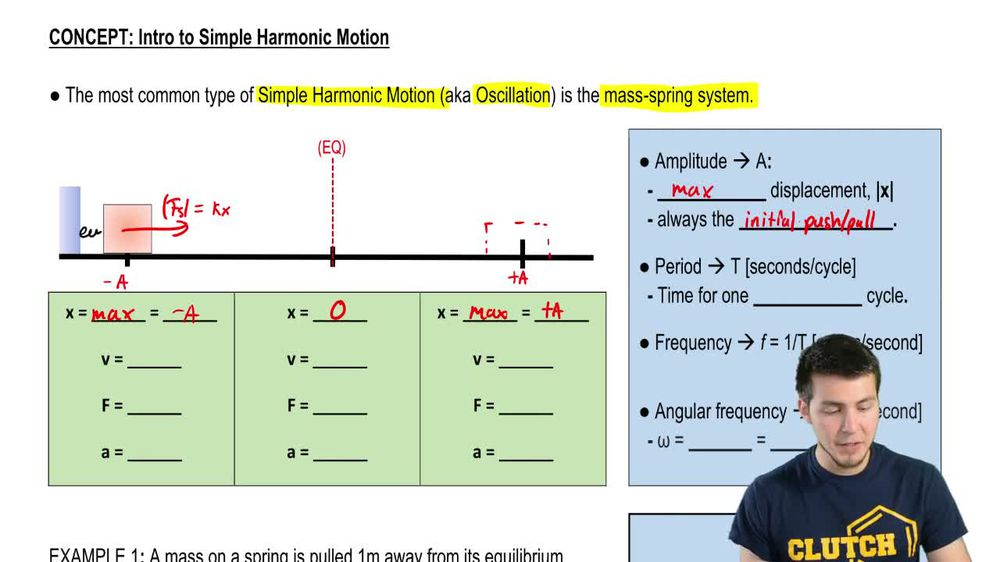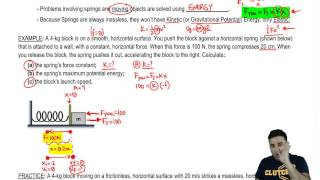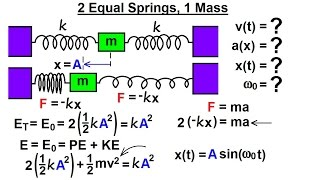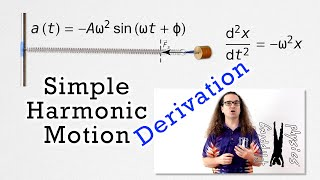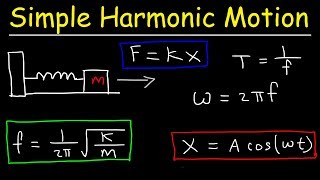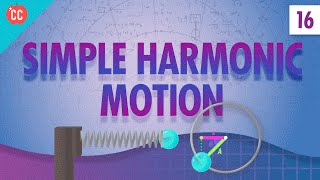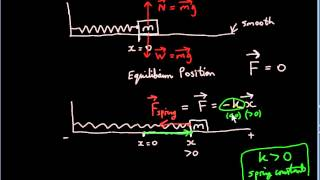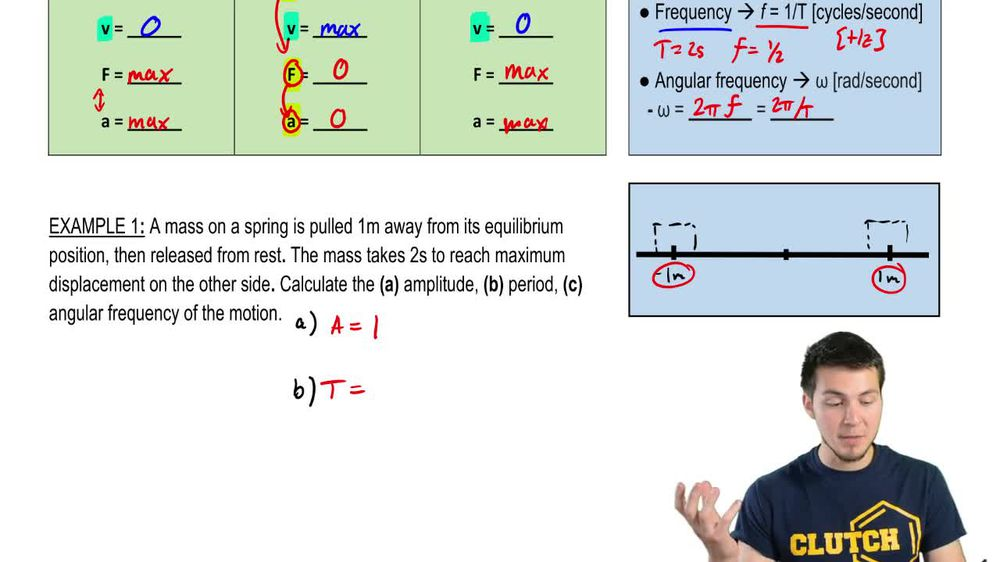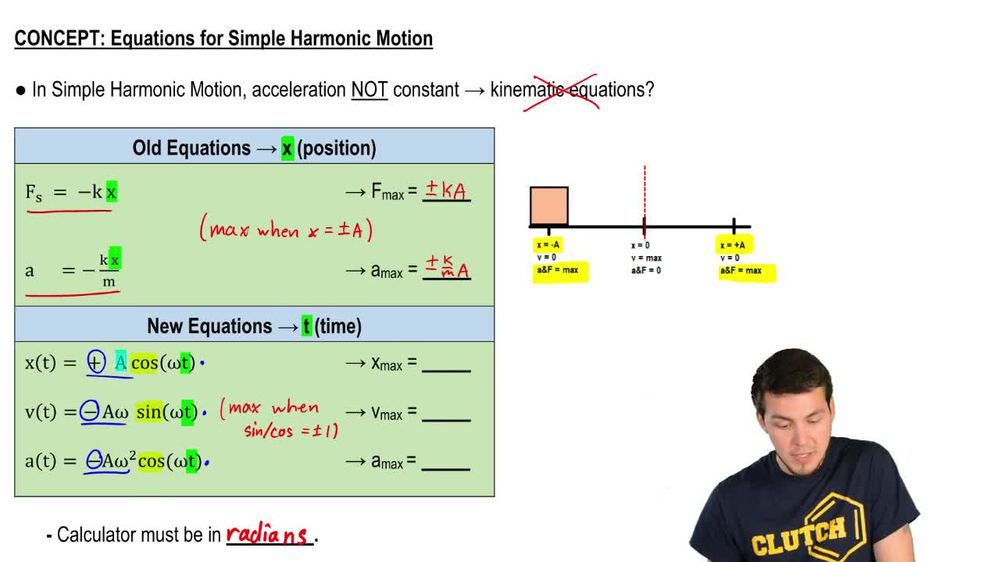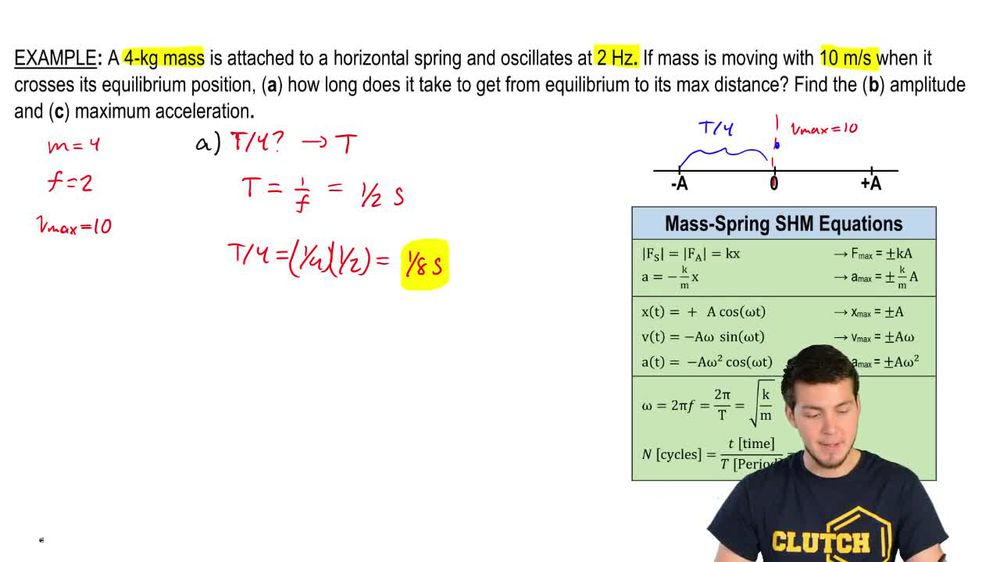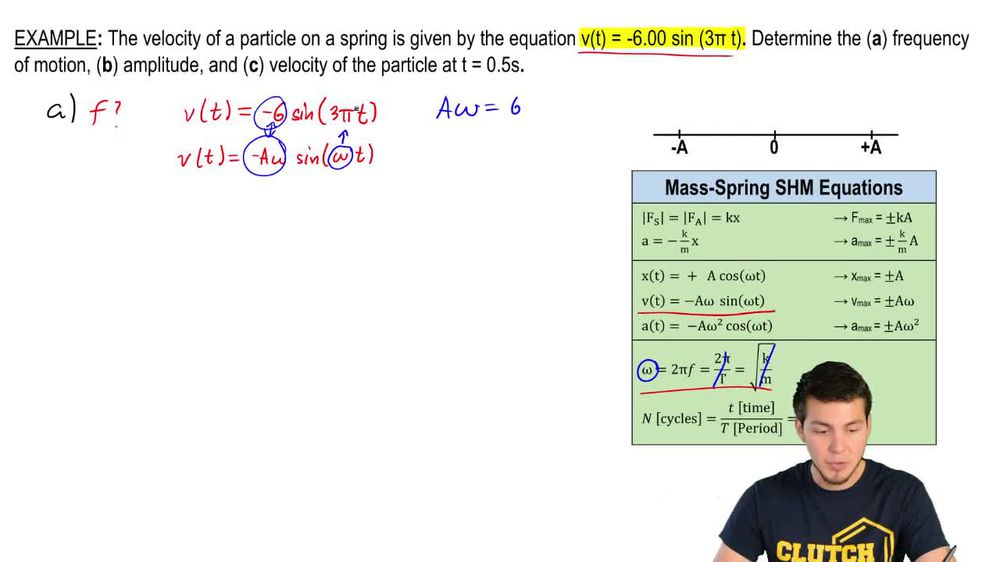Start typing, then use the up and down arrows to select an option from the list.
1. 17. Periodic Motion2. Intro to Simple Harmonic Motion (Horizontal Springs)# Intro to Simple Harmonic Motion

by Patrick Ford
221 views
4
1
4
Hey, guys. So for this video, I'm gonna introduce the motion of simple harmonic motion, the position, velocity and acceleration. And I'm also gonna give you guys some variables and definitions of useful for the rest of the chapter. So let's get to it. So the most common type of simple harmonic motion that will study in this chapter, or sometimes you'll see this oscillation is the mass spring system. So that's where you attach some fast to some spring. So if I pull this thing all the way back here, right, So I pull this on a spring and I let it go, The only force that's gonna be acting on that thing is the Spring Force, and that's equal to K X, Right? So I'm gonna pull this thing back. I'm gonna let it go, and basically this thing is gonna go all the way back to the equilibrium position. But it's gonna overshoot it and then land on the other side. So it's gonna go like something like that. And so basically, after that, it's just gonna oscillate between those two points forever, right? Those maximum displacements here, So the displacement is maximum on the left side, here and on the right side, right here. And so that the equilibrium position, We know that the X is equal to zero. So we call these two points on the outsides, the amplitude. So we've got plus a and minus a right here. And the amplitude is just the maximum displacement that this object has. It's always the initial push or pull that you apply against this object. So if you push it out to some distance and release it, that's the amplitude. So what is the velocity look like? When you do this, we're gonna pull this thing all the way back, and then you're just gonna let it go from rest. So that means that at this point, right here at the amplitude, the velocity is equal to zero. So then what happens is this thing starts speeding up faster and faster and faster goes through the equilibrium position. And then when it gets to the other side right here, it has to stop and then turn around. So that means that the velocity at this other amplitude is also equal to zero. And then what happens is as it's going back through here, the velocity is maximum here, and then it just keeps on doing that over and over again. So we have maximum velocity at the center, and so does this whole entire cycle over and over again. So the two variables that are related to that are the period and the frequency. The period, which is the letter T is the time that it takes for you to complete one complete cycle for you. Finish one complete cycle, and what's related to that variable is the frequency. So these things are just related by in verses of each other. So you see that the frequency is just one divided by T, So if you ever have one of them, you can get to the other one. So, for example, if I have a T that's equals, like two seconds on the frequency is just gonna be equal to one half of a cycle per second. And the units for that are gonna be in hurts now. Another variable that's related to the frequency. But not the same thing is the angular frequency. That's the letter. Omega. It's the units are rats, Radiance per second and all Omega is is just the frequency times two pi. We could also write it in a different way because the period and the frequency are in verses of each other. Okay, so what are the forces look like? A these three points. So when I pull it all the way back, the spring wants to push harder and harder, harder against me. So at this maximum displacement here that that means the force is also going to be maximum, Right? So take a look at K X if X is maximum that that means that F s also has to be maximum. And so we know that the maximum forces are gonna be here a the end points. And because of the equilibrium position, the X is equal to zero. Then that means that the force is equal to zero. Now, what about the acceleration? Will the acceleration and the force are related just by f equals m A. So if f is Max here, then that means the acceleration is Max here and here. And if f equals zero, then that means a is equal 20 So it looks like X f and A are all related to each other, and they're all in sync. So we've got X is all is zero F zero and a zero in the middle, whereas the weird one is this velocity here. So that's maximum when the other ones are zero. So let's take a look at an example here and see what we can find out.